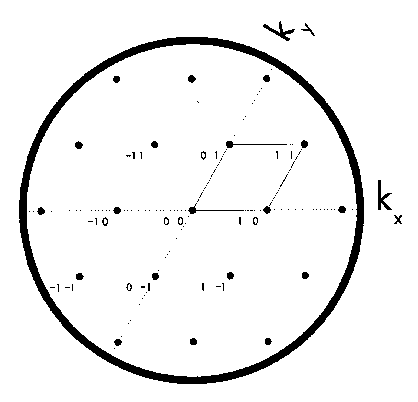Figure 2.2: Schematic LEED pattern for Si{111}1x1. Closed circles represent the diffracted beams for which the indices are noted. Reciprocal lattice directions and mesh are indicated. On a typical LEED screen, this would correspond to a 100eV pattern.

• Figure 2.1: Schematic LEED-AES retarding-field analyzer (RFA).
• Figure 2.2: Schematic LEED pattern for Si{111} 1x1.
• Figure 2.3: Schematic LEED pattern for Si{111}rt3*rt3-30-X.
• Figure 2.4: A LEED pattern of Si{111}7*7, at 100 eV.
• Figure 2.5: Schematic LEED Data Acquisition System.
• Figure 2.6: Reliability-factor (R_VHT, r_ZJ, and R_P) contour plots for the surface of TB{11-20}.
• Figure 2.7: Schematic AES Data Acquisition System.
• Figure 2.8: Typical Auger electron spectrum for Au deposited upon a Si{111} surface.
• Figure 2.9: Schematic LEED-AES Surface Analysis UHV System.